# 工程數學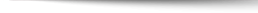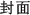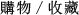+作者：

### Zill

+年份：
2022 年7 版
+ISBN：
9781284240771
+書號：
MA0481PC
+規格：

+頁數：
1064
+出版商：
Jones & Bartlett

\$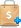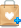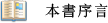The seventh edition of Advanced Engineering Mathematics provides learners with a modern and comprehensive compendium of topics that are most often covered in courses in engineering mathematics, and is extremely flexible to meet the unique needs of courses ranging from ordinary differential equations, to vector calculus, to partial differential equations.
Acclaimed author, Dennis G. Zill's  accessible writing style and strong pedagogical aids, guide students through difficult concepts with thoughtful explanations, clear examples, interesting applications, and contributed project problems.
Each new print copy includes a Navigate Companion Website with access to interactive and informative learning resources that gauge understanding and help students study more effectively.●Application problems such as Air Exchange, Potassium-40 Decay, Temperature of a Fluid, and more
●New section: Finite Fourier Transforms
●New examples and new problems (conceptual and applied)
●New figures and photos
●New Appendix A: Integral-Defined Functions
●Expanded table of Laplace in Appendix C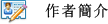Dennis Zill received a PhD in Applied Mathematics from Iowa State University, and is a former professor of Mathematics at Loyola Marymount University in Los Angeles, Loras College in Iowa, and California Polytechnic State University. He is also the former chair of the Mathematics department at Loyola Marymount University, where he currently holds a rank as Professor Emeritus of Mathematics. Zill holds interests in astronomy, modern literature, music, golf, and good wine, while his research interests include Special Functions, Differential Equations, Integral Transformations, and Complex Analysis.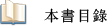Chapter  1  Introduction to Differential Equations
Chapter  2  First-Order Differential Equations
Chapter  3  Higher-Order Differential Equations
Chapter  4  The Laplace Transform
Chapter  5  Series Solutions of Linear Equations
Chapter  6  Numerical Solutions of Ordinary Differential Equations
Chapter  7  Vectors
Chapter  8  Matrices
Chapter  9  Vector Calculus
Chapter  10  Systems of Linear Differential Equations
Chapter  11  Systems of Nonlinear Differential Equations
Chapter  12  Fourier Series
Chapter  13  Boundary-Value Problems in Rectangular Coordinates
Chapter  14  Boundary-Value Problems in Other Coordinate Systems
Chapter  15  Integral Transforms
Chapter  16  Numerical Solutions of Partial Differential Equations
Chapter  17  Functions of a Complex Variable
Chapter  18  Integration in the Complex Plane
Chapter  19  Series and Residues
Chapter  20  Conformal Mappings